• 2022-04-19 12:35:08

# Python Pandas缺失值处理

在一些数据分析业务中，数据缺失是我们经常遇见的问题，缺失值会导致数据质量的下降，从而影响模型预测的准确性，这对于机器学习和数据挖掘影响尤为严重。因此妥善的处理缺失值能够使模型预测更为准确和有效。

## 为什么会存在缺失值？

前面章节的示例中，我们遇到过很多 NaN 值，关于缺失值您可能会有很多疑问，数据为什么会丢失数据呢，又是从什么时候丢失的呢？通过下面场景，您会得到答案。

其实在很多时候，人们往往不愿意过多透露自己的信息。假如您正在对用户的产品体验做调查，在这个过程中您会发现，一些用户很乐意分享自己使用产品的体验，但他是不愿意透露自己的姓名和联系方式；还有一些用户愿意分享他们使用产品的全部经过，包括自己的姓名和联系方式。因此，总有一些数据会因为某些不可抗力的因素丢失，这种情况在现实生活中会经常遇到。

## 什么是稀疏数据？

稀疏数据，指的是在数据库或者数据集中存在大量缺失数据或者空值，我们把这样的数据集称为稀疏数据集。稀疏数据不是无效数据，只不过是信息不全而已，只要通过适当的方法就可以“变废为宝”。

稀疏数据的来源与产生原因有很多种，大致归为以下几种：

由于调查不当产生的稀疏数据；

由于天然限制产生的稀疏数据；

文本挖掘中产生的稀疏数据。

## 缺失值处理

那么 Pandas 是如何处理缺失值的呢，下面让我们一起看一下。

import pandas as pd
import numpy as np
df = pd.DataFrame(np.random.randn(5, 3), index=['a', 'c', 'e', 'f','h'])
df = df.reindex(['a', 'b', 'c', 'd', 'e', 'f', 'g', 'h'])
print(df)


输出结果：

          0         1         2
a  0.187208 -0.951407  0.316340
b       NaN       NaN       NaN
c -0.365741 -1.983977 -1.052170
d       NaN       NaN       NaN
e -1.024180  1.550515  0.317156
f -0.799921 -0.686590  1.383229
g       NaN       NaN       NaN
h -0.207958  0.426733 -0.325951


上述示例，通过使用 reindex（重构索引），我们创建了一个存在缺少值的 DataFrame 对象。

## 检查缺失值

为了使检测缺失值变得更容易，Pandas 提供了 isnull() 和 notnull() 两个函数，它们同时适用于 Series 和 DataFrame 对象。

import pandas as pd
import numpy as np
df = pd.DataFrame(np.random.randn(5, 3), index=['a', 'c', 'e', 'f','h'])
df = df.reindex(['a', 'b', 'c', 'd', 'e', 'f', 'g', 'h'])
print(df['noe'].isnull())


输出结果：

a    False
b     True
c    False
d     True
e    False
f    False
g     True
h    False
Name: 1, dtype: bool


notnull() 函数，使用示例：

import pandas as pd
import numpy as np
df = pd.DataFrame(np.random.randn(5, 3), index=['a', 'c', 'e', 'f','h'])
df = df.reindex(['a', 'b', 'c', 'd', 'e', 'f', 'g', 'h'])
print df['one'].notnull()


输出结果：

a     True
b    False
c     True
d    False
e     True
f     True
g    False
h     True
Name: 1, dtype: bool


## 缺失数据计算

计算缺失数据时，需要注意两点：首先数据求和时，将 NA 值视为 0 ，其次，如果要计算的数据为 NA，那么结果就是 NA。示例如下：

import pandas as pd
import numpy as np
df = pd.DataFrame(np.random.randn(5, 3), index=['a', 'c', 'e', 'f','h'],columns=['one', 'two', 'three'])
df = df.reindex(['a', 'b', 'c', 'd', 'e', 'f', 'g', 'h'])
print (df['one'].sum())
print()


输出结果：

3.4516595395128


## 清理并填充缺失值

Pandas 提供了多种方法来清除缺失值。fillna() 函数可以实现用非空数据“填充”NaN 值。

1) 用标量值替换NaN值

下列程序将 NaN 值 替换为了 0，如下所示：

import pandas as pd
import numpy as np
df = pd.DataFrame(np.random.randn(3, 3), index=['a', 'c', 'e'],columns=['one',
'two', 'three'])
df = df.reindex(['a', 'b', 'c'])
print(df)
#用 0 填充 NaN
print (df.fillna(0))


输出结果：

     one       two     three
a  1.497185 -0.703897 -0.050513
b       NaN       NaN       NaN
c  2.008315  1.342690 -0.255855

one       two     three
a  1.497185 -0.703897 -0.050513
b  0.000000  0.000000  0.000000
c  2.008315  1.342690 -0.255855


当然根据您自己的需求，您也可以用其他值进行填充。

2) 向前和向后填充NA

在前一节，我们介绍了 ffill() 向前填充和 bfill() 向后填充，使用这两个函数也可以处理 NA 值。示例如下：

import pandas as pd
import numpy as np
df = pd.DataFrame(np.random.randn(5, 3), index=['a', 'c', 'e', 'f','h'],columns=['one', 'two', 'three'])
df = df.reindex(['a', 'b', 'c', 'd', 'e', 'f', 'g', 'h'])
print df.fillna(method='ffill')


输出结果：

        one       two     three
a  0.871741  0.311057  0.091005
b  0.871741  0.311057  0.091005
c  0.107345 -0.662864  0.826716
d  0.107345 -0.662864  0.826716
e  1.630221  0.482504 -0.728767
f  1.283206 -0.145178  0.109155
g  1.283206 -0.145178  0.109155
h  0.222176  0.886768  0.347820


或者您也可以采用向后填充的方法。

3) 使用replace替换通用值

在某些情况下，您需要使用 replace() 将 DataFrame 中的通用值替换成特定值，这和使用 fillna() 函数替换 NaN 值是类似的。示例如下：

import pandas as pd
import numpy as np
df = pd.DataFrame({'one':[10,20,30,40,50,666], 'two':[99,0,30,40,50,60]})
#使用replace()方法
print (df.replace({99:10,666:60,0:20}))


输出结果：

   one  two
0   10   10
1   20   20
2   30   30
3   40   40
4   50   50
5   60   60


## 删除缺失值

如果想删除缺失值，那么使用 dropna() 函数与参数 axis 可以实现。在默认情况下，按照 axis=0 来按行处理，这意味着如果某一行中存在 NaN 值将会删除整行数据。示例如下：

import pandas as pd
import numpy as np
df = pd.DataFrame(np.random.randn(5, 3), index=['a', 'c', 'e', 'f','h'],columns=['one', 'two', 'three'])
df = df.reindex(['a', 'b', 'c', 'd', 'e', 'f', 'g', 'h'])
print(df)
#删除缺失值
print (df.dropna())


输出结果：

        one       two     three
a -2.025435  0.617616  0.862096
b       NaN       NaN       NaN
c -1.710705  1.780539 -2.313227
d       NaN       NaN       NaN
e -2.347188 -0.498857 -1.070605
f -0.159588  1.205773 -0.046752
g       NaN       NaN       NaN
h -0.549372 -1.740350  0.444356

one       two     three
a -2.025435  0.617616  0.862096
c -1.710705  1.780539 -2.313227
e -2.347188 -0.498857 -1.070605
f -0.159588  1.205773 -0.046752
h -0.549372 -1.740350  0.444356


axis = 1 表示按列处理，处理结果是一个空的 DataFrame 对象。

更多相关内容
• 1.决策树缺失值处理 1.1对于训练模型，训练数据部分样本的部分特征值缺失的情况下，做法是：把该数据按比例分成三份数据。可以算出该特征的信息增益。 1.2对于预测数据，C4.5中采用的方法是：测试样本在该属性值上有...
• 缺失值处理 缺失值首先需要根据实际情况定义 可以采取直接删除法 有时候需要使用替换法或者插值法 常用的替换法有均值替换、前向、后向替换和常数替换 import pandas as pd import numpy as np import os os.getcwd...
• R语言处理缺失值处理数据过程中，避免不了会产生一些缺失值，如未填写数据或者编码错误等原因，用NA表示缺失值。在R语言中，is.na()函数可以判断元素是否是缺失值，从而返回逻辑值(TRUE/FALSE)，所以该函数将会...r语言
• 本文介绍通过拉格朗日插值法进行缺失值的填充： 先用一组简单的数据看一下拉格朗日插值法 from scipy.interpolate import lagrange import matplotlib.pyplot as plt import numpy as np %matplotlib inline plt....
• 主要介绍了python 缺失值处理的方法（Imputation），文中通过示例代码介绍的非常详细，对大家的学习或者工作具有一定的参考学习价值，需要的朋友们下面随着小编来一起学习学习吧
• 首先是缺失值处理。 #读取数据 import pandas as pd filepath= 'F:/...'#本地文件目录 df= pd.read_csv(train,sep=',')#df数据格式为DataFrame 查看缺失值 查看每一特征是否缺失及缺失值数量可能影响着处理缺失值的...
• 主要给大家介绍了关于Python Pandas对缺失值处理方法，文中通过示例代码介绍的非常详细，对大家学习或者使用Python Pandas具有一定的参考学习价值，需要的朋友们下面来一起学习学习吧
• 因近期进行时间序列分析时遇到了数据预处理中的缺失值处理问题，其中日期缺失和填充在网上没有找到较好较全资料，耗费了我一晚上工作时间，所以下面我对这次时间序列缺失值处理学习做了以下小结以供之后同行们参考...
• 但是如果缺失值占比比较大，这种直接删除缺失值处理方法就会丢失重要信息。 直接删除法处理缺失值时，需要检测样本总体中缺失值的个数。Python中统计缺失值的方法如下（下面结合具体数据集，直接上代码）： import...
• 该Eviews文件对应我的博客（平衡面板数据中的缺失值可以存在吗？https://blog.csdn.net/zhouxiaojieaaa/article/details/110999819）
• 缺失值（missing data）又叫不完整数据，其发生的原因主要分为两类，一类是个人填写疏忽、题意不明漏答、拒绝作答等内部因素，另一种是数据输入所造成的失误等外部因素。 缺失值最大的影响在于造成样本的流失，同时...
• 入门级基础知识，本人学习整理笔记，对小白很友好，有兴趣的学习的朋友可以查阅。
• 数学建模时对数据进行预处理，用移动平均法对缺失值进行填充
• 通过Long Short-Term Memory长短期记忆网络处理时间序列缺失值问题。
• 常见的缺失值处理方式有，过滤、填充。 一、缺失值的判断 pandas使用浮点值NaN(Not a Number)表示浮点数和非浮点数组中的缺失值，同时python内置None值也会被当作是缺失值。 a、Series的缺失值判断 s = Series([a...
• 5、将姓名的缺失值进行填充 6、将清洗好的数据保存到指定excel中 原始数据： 最后输出数据： 处理步骤： 1、读取数据： 2、删除全是空值的列 3、删除全是空值的行 4、将分数列值为NAN（空值）的填充为0分 5、...
• 不论是自己爬虫获取的还是从公开数据源上获取的数据集，都不能保证数据集是完全准确的，难免会有一些缺失值。而以这样数据集为基础进行建模或者数据分析时，缺失值会对结果产生一定的影响，所以提前...

不论是自己爬虫获取的还是从公开数据源上获取的数据集，都不能保证数据集是完全准确的，难免会有一些缺失值。而以这样数据集为基础进行建模或者数据分析时，缺失值会对结果产生一定的影响，所以提前处理缺失值是十分必要的。

对于缺失值的处理大致可分为以下三方面：

• 不处理

• 删除含有缺失值的样本

• 填充缺失值

不处理应该是效果最差的了，删除虽然可以有效处理缺失值，但是会损伤数据集，好不容易统计的数据因为一个特征的缺失说删就删实在说不过去。填充缺失值应该是最常用且有效的处理方式了，下面介绍四种处理缺失值的常用Tips。

我自己构建了一个简易的含有缺失值的DataFrame，所有操作都基于这个数据集进行。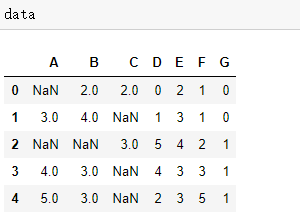## 1、删除缺失值

删除虽说是一个可行的方式，但肯定是不能随便删除的，比如一个样本中仅有一个特征的值缺失，这样的情况下填充取得的效果一定会优于删除，所以在删除缺失值时，我们需要一个衡量的标准。

删除的方式无非有两种，一是删除缺失值所在行，也就是含有缺失值的样本；二就是删除缺失值所在列，也就是含有缺失值的特征，下面以后者为例。

首先需要确定的是删除的标准是什么？比如一个特征的缺失值所占比例已经超过了50%，如果选择填充的话，就表明该特征超五成的值都是自己猜测填入的，导致误差可能比删除这个特征还要大。

def find_missing(data):
#统计缺失值个数
missing_num = data.isna().sum(axis=0).sort_values(ascending=False)
missing_prop = missing_num/float(len(data)) #计算缺失值比例
drop_index = missing_prop[missing_prop>0.5].index.tolist() #过滤要删除特征名
return drop_index



在确定了这个标准之后，就可以利用一个自定义函数，将我们期望实现的功能封装至函数中。比如上面这个函数，先确定每个特征的缺失值个数并降序排列，然后计算缺失值比例，最后利用布尔索引得到需要删除的特征名。

data2 = data.copy()
data2.drop(find_missing(data2),axis = 1)



在数据集上应用这个函数，可以看到缺失值占比超50%的特征C被删除了。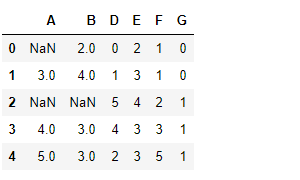这个衡量标准自己可以依据情况设定，然后删除样本的方式可以类比上述删除特征的方式。

## 2、pandas填充

pandas中的fillna()应该是最常用的一种填充缺失值方法，可以指定填充指定列或者整个数据集。

data['A'].fillna(value = data['A'].mean(),limit=1)



比如上面这句代码，就是只填充特征A一列，填充的选择可以利用平均数、中位数、众数等等，limit是限制要填充的个数，如果有两个缺失值，但是参数limit=1的话，按顺序填充第一个。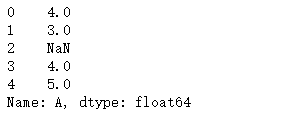value参数也允许传入字典格式，键为要填充的特征名，值为要填充的缺失值。

values = {'A':4,'B':3,'C':4}
data.fillna(value=values)



填充之后结果如下：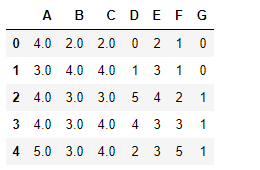fillna()方法固然简单，但前提是含有缺失值的特征比较少，如果很多的话，代码就会很冗杂，客观性也比较差。

## 3、sklearn填充

第二种填充方式是利用sklearn中自带的API进行填充。

from sklearn.impute import SimpleImputer
data1 = data.copy()
#得到含有缺失值的特征
miss_index = data1.isna().any()[data1.isna().any().values == True].index.tolist()
print(miss_index)
'''
['A', 'B', 'C']
'''



首先利用布尔索引得到数据集含有缺失值的特征，后续操作只针对含有缺失值的特征。

miss_list = []
for i in miss_index:
#将一维数组转化为二维
miss_list.append(data1[i].values.reshape(-1,1))
for i in range(len(miss_list)):
#利用众数进行填充
imp_most = SimpleImputer(strategy='most_frequent')
imp_most = imp_most.fit_transform(miss_list[i])
data1.loc[:,miss_index[i]] = imp_most



最需要注意的一点是SimpleImputer传入的参数至少要是二维，如果将直接索引出的一列特征传入的话，是会发生报错的，所以必须利用reshape()将一维转化为二维。之后的操作就是先实例化、然后训练模型，最后用填充后的数据覆盖之前的数据。

参数strategy共有四个选项可填：

• 1、mean:平均数

• 2、median:中位数

• 3、most_frequent:众数

• 4、constant：如果参数指定这个，将会选择另一个参数fill_value中的值作为填充值。

SimpleImputer优于fillna()之处在于前者可以一行语句指定填充值的形式，而利用fillna()需要多行重复语句才能实现，或者需要提前计算某列的平均值、中位数或者众数。

## 4、利用算法填充

我们都知道一般的算法建模是通过n个特征来预测标签变量，也就是说特征与标签标量之间存在某种关系，那么通过标签变量与(n-1)个特征是否能预测出剩下的一个特征呢？答案肯定是可以的。

实际上标签变量和特征之间可以相互转化，所以利用这种方法就可以填补特征矩阵中含有缺失值的特征，尤其适用于一个特征缺失值很多，其余特征数据很完整，特别标签变量那一列的数据要完整。

但是往往一个特征矩阵中很多特征都含有缺失值，对于这种情况，可以从特征缺失值最少的一个开始，因为缺失值越少的特征需要的信息也就越少。

当预测一个特征时，其余特征的缺失值都需要用0暂时填补，每当预测完一列特征，就用预测出的结果代替原数据集对应的特征，然后预测下一特征，直至最后一个含有缺失值的特征，此时特征矩阵中应该没有需要利用0填补的缺失值了，表示数据集已经完整。

以随机森林算法为例，实现上面表述填充缺失值的过程。

data3 = data.copy()
#获取含有缺失值的特征
miss_index = data3.isna().any()[data3.isna().any().values == True].index.tolist()
#按照缺失值多少，由小至大排序，并返回索引
sort_miss_index = np.argsort(data3[miss_index].isna().sum(axis = 0)).values
sort_miss_index
'''
array([1, 0, 2], dtype=int64)
'''



第一步就是通过布尔索引得到含有缺失值的特征，并且根据缺失值的多少进行由小到大排序，这里选择利用argsort，因为返回的排序是特征在特征矩阵中的索引。

for i in sort_miss_index:
data3_list =  data3.columns.tolist() #特征名
data3_copy = data3.copy()
fillc = data3_copy.iloc[:,i] #需要填充缺失值的一列
# 从特征矩阵中删除这列，因为要根据已有信息预测这列
df = data3_copy.drop(data3_list[i],axis = 1)
#将已有信息的缺失值暂用0填补
df_0 = SimpleImputer(missing_values=np.nan,strategy='constant',fill_value=0).fit_transform(df)

Ytrain = fillc[fillc.notnull()]#训练集标签为填充列含有数据的一部分
Ytest = fillc[fillc.isnull()]#测试集标签为填充列含有缺失值的一部分

Xtrain = df_0[Ytrain.index,:]#通过索引获取Xtrain和Xtest
Xtest = df_0[Ytest.index,:]

rfc = RandomForestRegressor(n_estimators = 100)#实例化
rfc = rfc.fit(Xtrain,Ytrain)  # 导入训练集进行训练
Ypredict = rfc.predict(Xtest) # 将Xtest传入predict方法中，得到预测结果
#获取原填充列中缺失值的索引
the_index = data3[data3.iloc[:,i].isnull()==True].index.tolist()
data3.iloc[the_index,i] = Ypredict# 将预测好的特征填充至原始特征矩阵中



这部分代码主要的思想就是，先将需预测的一列特征暂定为标签，然后预测列中含有数据的一部分作为训练集，含有缺失值的一部分作为测试集，通过随机森林在训练集上建模，利用模型在测试集的基础上得到缺失值那部分的数据，最后填充值原特征矩阵中。

最后预测出的结果如下：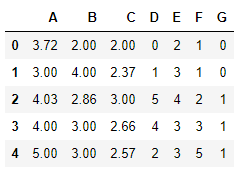可以看到原特征矩阵中缺失值的一部分被填充好了，这种利用算法填充缺失值的方法应该是精度最高的，因为缺失值是在原有数据的基础上预测出的，而不是随意猜测的，但缺点就是没有前几种便利，当特征或缺失值较多时会比较耗时。

## 说在最后

缺失值处理是特征工程至关重要的一步，而特征工程和数据本身往往决定着一个模型的上限，所以数据集中的缺失值在一个项目中值得我们花些时间去处理，而不是用自己的幸运数字随意填充，一句话总结就是"你不要你觉得，而是模型觉得"。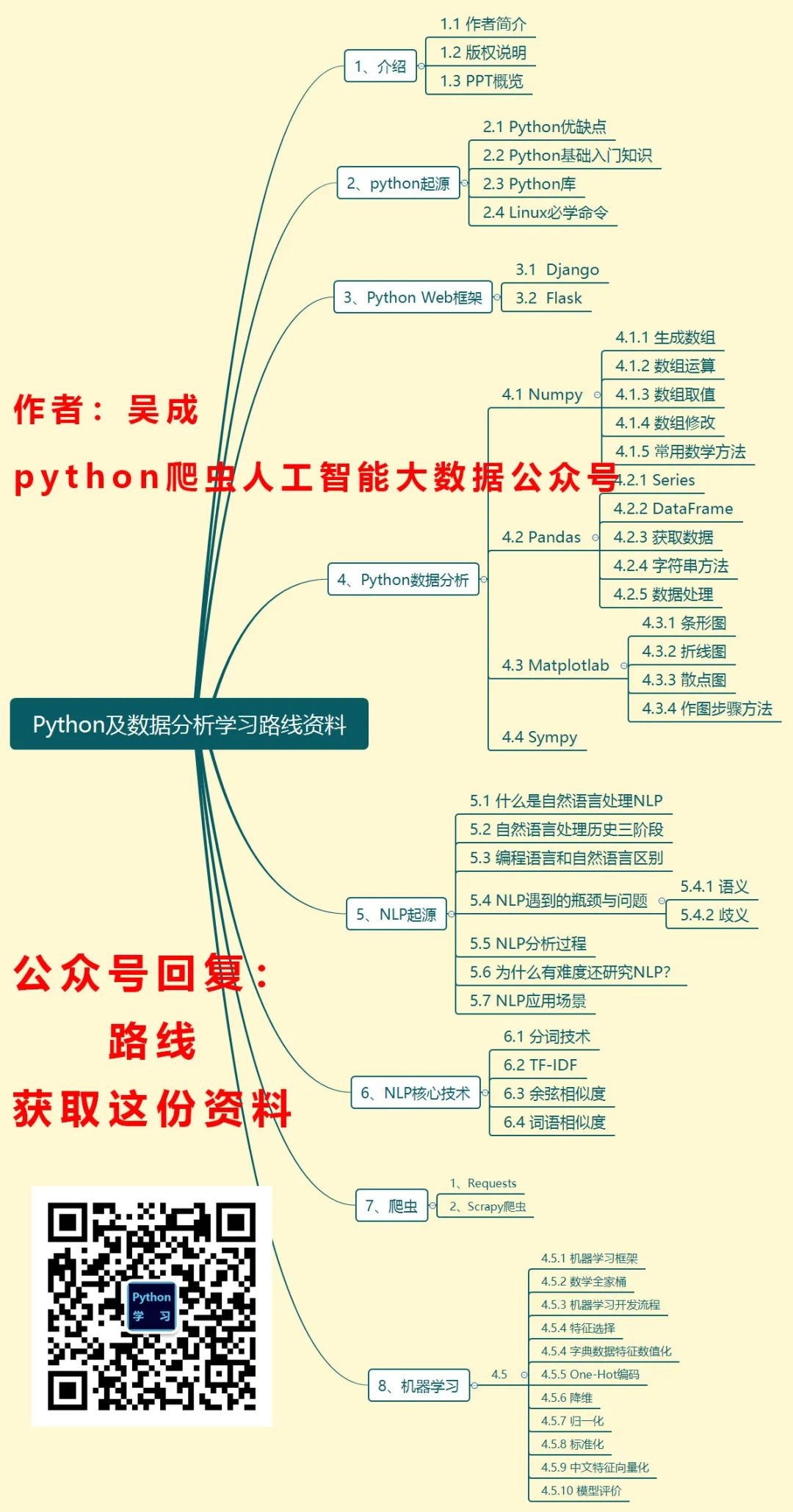展开全文算法 python 机器学习 数据挖掘
• 今天小编就为大家分享一篇python实现数据清洗(缺失值与异常值处理)，具有很好的参考价值，希望对大家有所帮助。一起跟随小编过来看看吧
•笔记
• 对于数值型数据，分为缺失值（NAN）和非缺失值对于缺失值的检测，可以通过Python中pandas库的Series类对象的isnull方法进行检测。 import pandas as pd import numpy as np string_data = pd.Series(['Benzema',...
• 缺失值：在dataframe中为nan或者naT（缺失时间），在series中为none或者nan即可 3.函数具体解释 DataFrame.dropna(axis=0, how=’any’, thresh=None, subset=None, inplace=False) 函数作用：删除含有空值的行或列 ...
• 该Eviews文件对应我的博客-（平衡面板数据中的缺失值可以存在吗？https://www.jianshu.com/p/39d2f529237b）
• 主要介绍缺失值的定义、产生与处理，对缺失值处理时的一些影响...